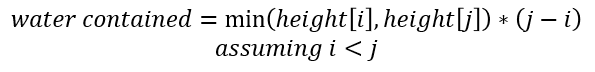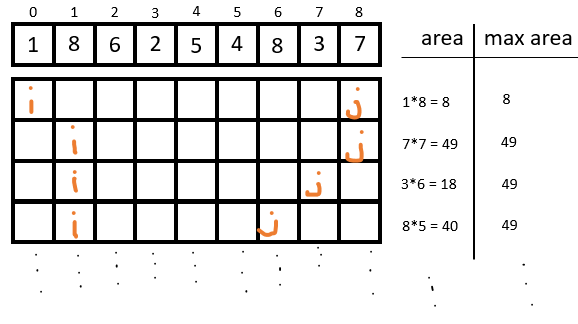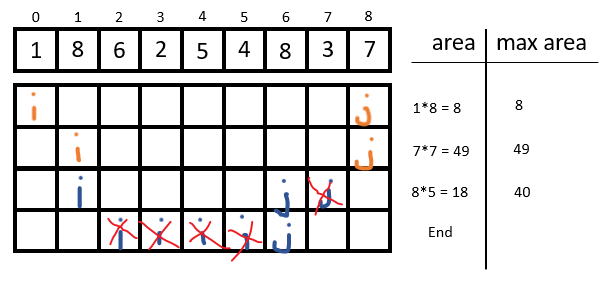# Leetcode 11 — Container With Most Water

This article will cover and explain a solution to Leetcode 11, Container With Most Water.

# Problem Overview

You are given an integer array `height` of length `n`. There are `n` vertical lines drawn such that the two endpoints of the `i'th` line are `(i, 0)` and `(i, height[i])`.

Find two lines that together with the x-axis form a container, such that the container contains the most water.

Return the maximum amount of water a container can store. Notice that you may not slant the container.

Constraints:

`n == height.length`

`2 <= n <= 10^5`

`0 <= height[i] <= 10^4`

So, one question I have is if the vertical lines displace any water? Answer: No, they don’t. One clarification, if it’s not already obvious from the may not slant comment, the water must be level to the x-axis. In other words, if the height of the left side is 6 and the height of the right side is 8, the max height is 6. Let’s continue to an example!

# Example

`Input: height = [1, 8, 6, 2, 5, 4, 8, 3, 7]`

`output: 49`

There’s a bit going on in this chart so let me explain:

• The x-axis is the index of elements in `height`
• The y-axis is the height, as listed in `height`
• The dashed box calls out the largest container (the solution), also just an area, in this example
• The red bars represent the ‘vertical lines`, or edges, of the solution pair
• Circled in blue and reinforced with the curly brace is the width of the largest container; and below it you see the calculation for its area.
• In green, I highlighted what you may have considered the largest container, and ran through the area calculation to show it actually is not.

Hopefully that explains.

# Strategy, Concepts, and Solution

First off, let’s get on the same page of how much a water a container can contain:A simple calculation to determine the area of a rectangle

What does this tell us? It tells us that the larger the difference between `j` and `i` , the larger the area. If it’s not clear, let me explain like this: the minimum width of a container is 1, which means `j = i + 1, and ((i+1) — i) = 1` . So as `j` increases, so does the difference: `j = i + 3, and so ((i + 3) — i) = 3` . As a result, we’re multiplying whatever the height is by a larger number.

But why is that important? Because it tell us to be greedy with our width, and work outside to inside:

Now what about our height? Well, we want to be greedy about that too because if the height is larger, then the area is larger too! So, that brings us to how we’re going to iterate over this array. If `height[i] < height[j]` then we want to keep `j`(keep our container as wide as possible) and increment `i` . To get an idea, let’s jump right into how we’d iterate over this:Area is determined from the equation determined above. Max area tracks the largest area calculated thus far.

Starting from the top row with `i, j` we see that we calculated an area of `8` and updated the largest area as such (first round). Next, notice that `height[i]< height[j]` and as a result `i` is incremented in the next iteration. Continuing this pattern for one more round … we calculate an area of `49` for the new position `i, j` , update our largest area observed, and notice that `height[i] > height[j]` so we decrement `j`

BUT wait, notice that there’s a small optimization we can do to avoid unnecessary calculations:Optimized 2 pointer — Remove unnecessary checks

In blue is what is different from the first loop. Here, when we reduce the width (window) size in the step when we check which was a smaller height, we skip values until we find a new height that is greater than the previous. Why? The width is shrinking, so our area is too, and the only way for our area to grow is for our height to increase. As such, we will always have a smaller area as the width unless the height grows in some way. Looking at above again, we end quickly because when we increment `i` , we compare it to its previous largest height 8. We see 6 < 8, increment `i`, 2<8, increment `i`, 5<8, increment `i`, 4<8, increment `i`, `i is NOT < j` and we end because we’ve checked all possible areas.

These explanations should make this solution very easy to follow:

I hope you enjoyed the article :) !

--

--## Thomas Higginson

Software Engineer working on Cognitive EW capabilities, and human that enjoys making smiles. Welcome.